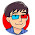## Thursday, July 5, 2018

### 3D Photos - Pink Flamingos

I used er9b to rectify/align the images.

er9b outputs the mean vertical disparity error and the min/max disparities which can be used as input to the depth map generators. I will typically use the min/max disparities given in the second line as it slightly expands the range given in the first line and gets rid of outliers.

Mean vertical disparity error = 0.256639
Min disp = -68 Max disp = 6
Min disp = -75 Max disp = 13

Note that, in this case, there is really not much difference between the input images and the rectified images. This means the input images were well aligned to begin with. Always a good thing.

I used dmag5 to get the depth map as it behaves the best, in general.

Input to dmag5:
min disparity for image 1 = -75
max disparity for image 1 = 13
disparity map for image 1 = depthmap_l.png
disparity map for image 2 = depthmap_r.png
occluded pixel map for image 1 = occmap_l.png
occluded pixel map for image 2 = occmap_r.png
radius = 16
alpha = 0.9
truncation (color) = 30
truncation (gradient) = 10
epsilon = 255^2*10^-4
disparity tolerance = 0
radius to smooth occlusions = 9
sigma_space = 9
sigma_color = 25.5
downsampling factor = 2

Note that I used the min/max disparities given by er9b in my input to dmag5.

I used dmag9b to improve the depth map.

Input to dmag9b:
sample_rate_spatial = 32
sample_rate_range = 8
lambda = 0.25
hash_table_size = 100000
nbr of iterations (linear solver) = 25
sigma_gm = 1
nbr of iterations (irls) = 32
radius (confidence map) = 12
gamma proximity (confidence map) = 12
gamma color similarity (confidence map) = 12
sigma (confidence map) = 32

If you want to further smooth out the disparities, you are welcome to use any of the edge preserving smoothers I have on offer. Maybe eps7 or eps9 would make things smoother. I don't think it's necessary to do more smoothing though.

I used wigglemaker to generate the 3d gif aka wigglegram aka wobbler.

Wigglemaker uses a single image and depth map to produce the wigglegram. If you want 3d gifs that make use of both images and depth maps (left and right), you can use fsg6. The advantage of using both images and depth maps is that there is virtually no inpainting.

#### 1 comment:

1.Thank you! Very good explanation page !